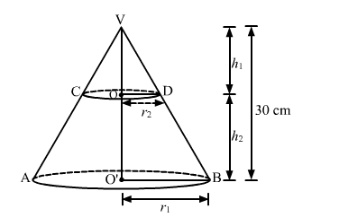# The height of a cone is 30 cm.`
Question:

The height of a cone is $30 \mathrm{~cm}$. A small cone is cut off at the top by a plane parallel to the base. If its volume be $\frac{1}{27}$ of the volume of the given cone, then the height above the base at which the section has been made, is

(a) 10 cm

(b) 15 cm

(c) 20 cm

(d) 25 cm

Solution:Let VAB be cone of height 30 cm and base radius r1 cm.

Suppose it is cut off by a plane parallel to the base at a height h2 from the base of the cone.

Clearly $\Delta V O D \sim \Delta V O^{\prime} B$

Therefore,

$\frac{O V}{O^{\prime} V}=\frac{O D}{O^{\prime} B}$

$\Rightarrow \frac{h_{1}}{30}=\frac{r_{2}}{r_{1}}$

But,

Volume of cone $V C D=\frac{1}{27}$ Volume of cone $V A B$

$\Rightarrow \frac{1}{3} \pi\left(\mathrm{r}_{2}\right)^{2} \mathrm{~h}_{1}=\frac{1}{27}\left(\frac{1}{3} \pi\left(\mathrm{r}_{1}\right)^{2} 30\right)$

$\Rightarrow\left(\frac{\mathrm{r}_{2}}{\mathrm{r}_{1}}\right)^{2} \mathrm{~h}_{1}=\frac{10}{9}$

$\Rightarrow\left(\frac{\mathrm{h}_{1}}{30}\right)^{2} \mathrm{~h}_{1}=\frac{10}{9}$

$\Rightarrow \mathrm{h}_{1}=10$Hence,

Required height

$=30-10$

$=20 \mathrm{~cm}$

Hence, the correct answer is choice (c).